• Fall 2021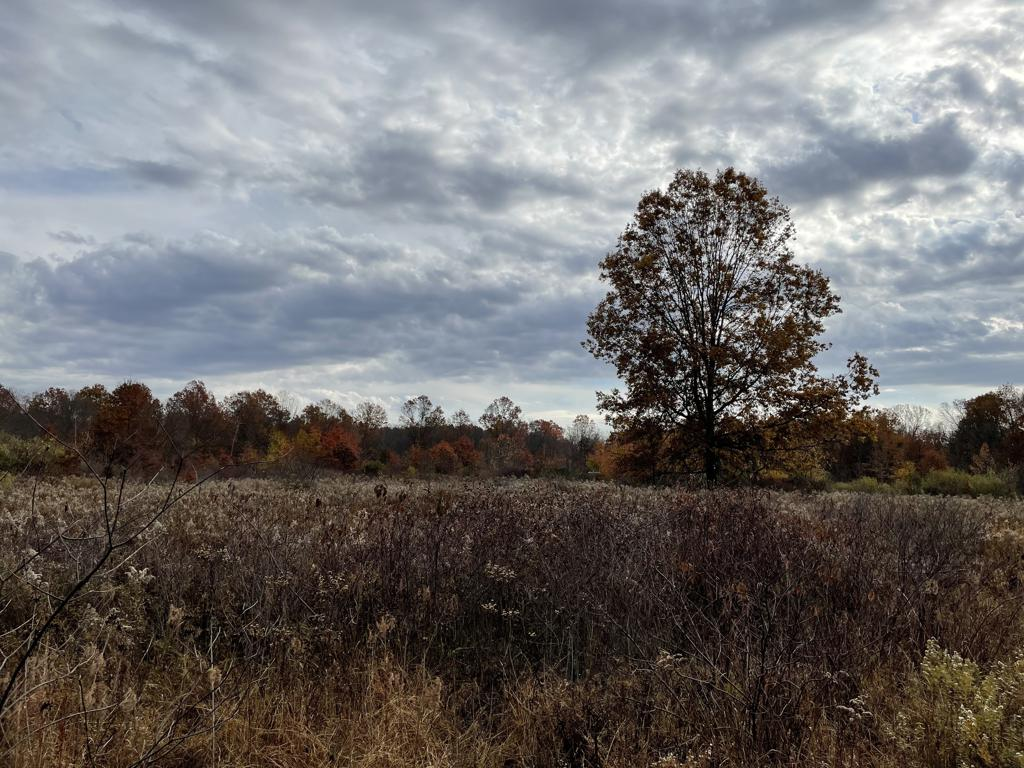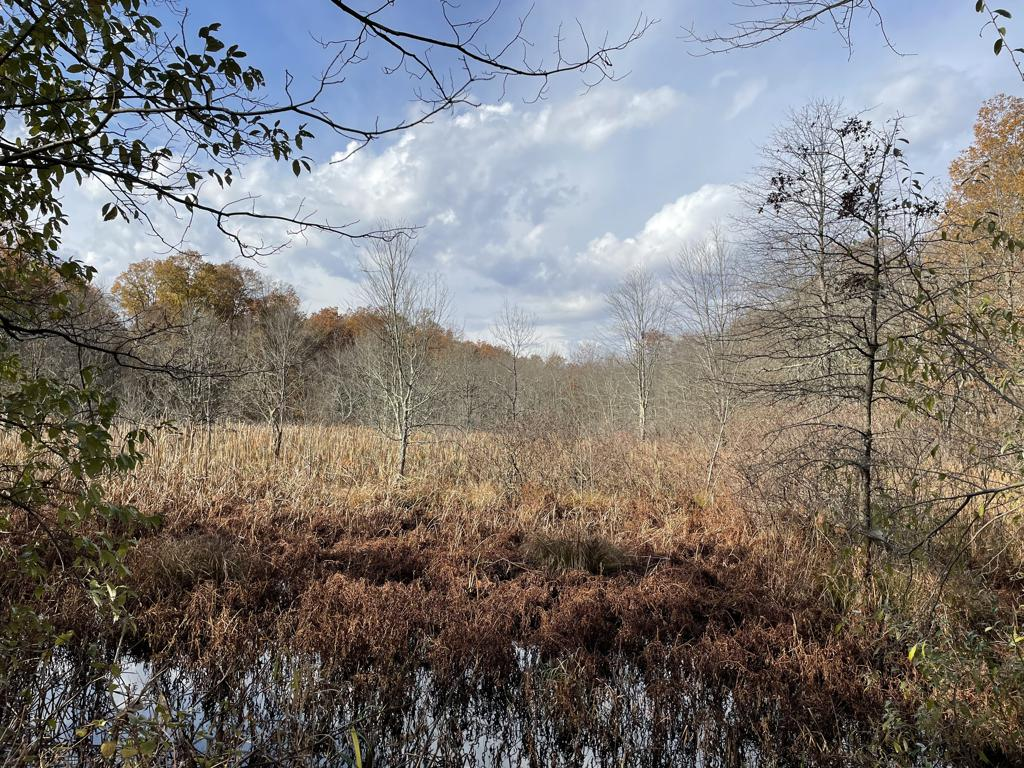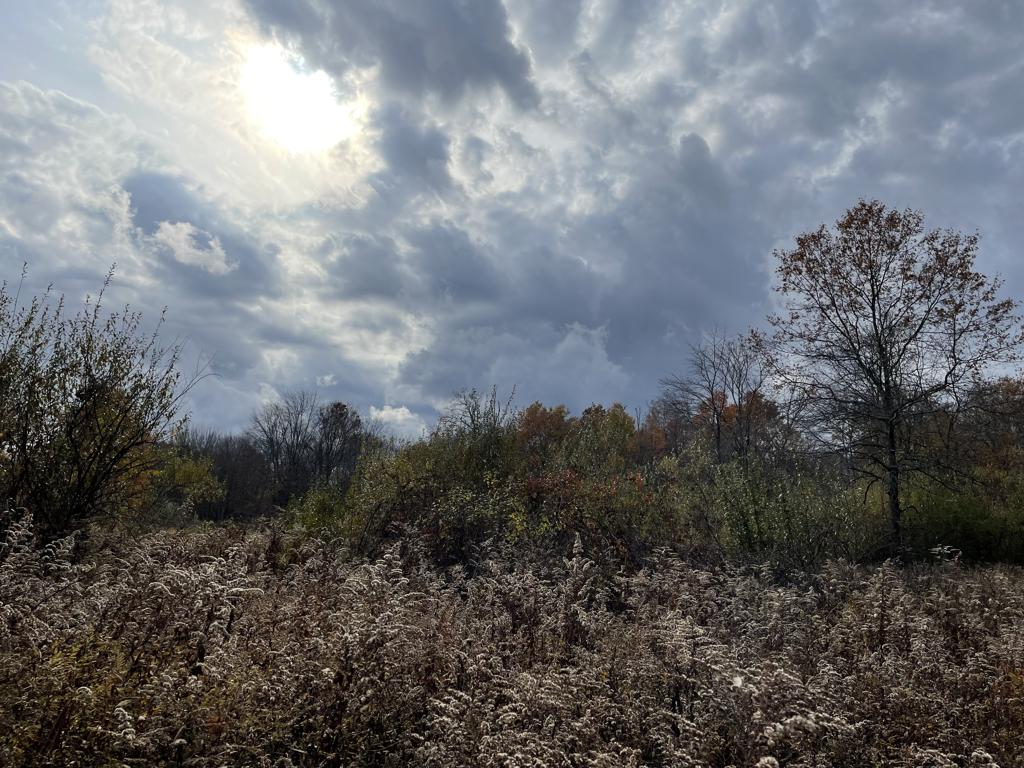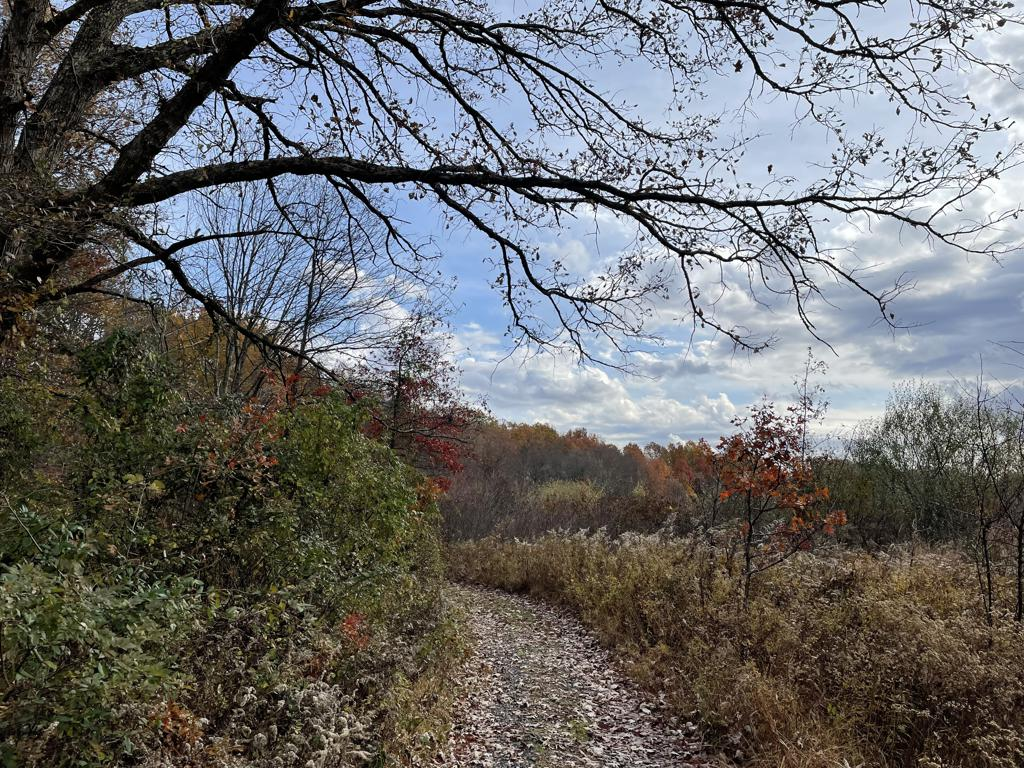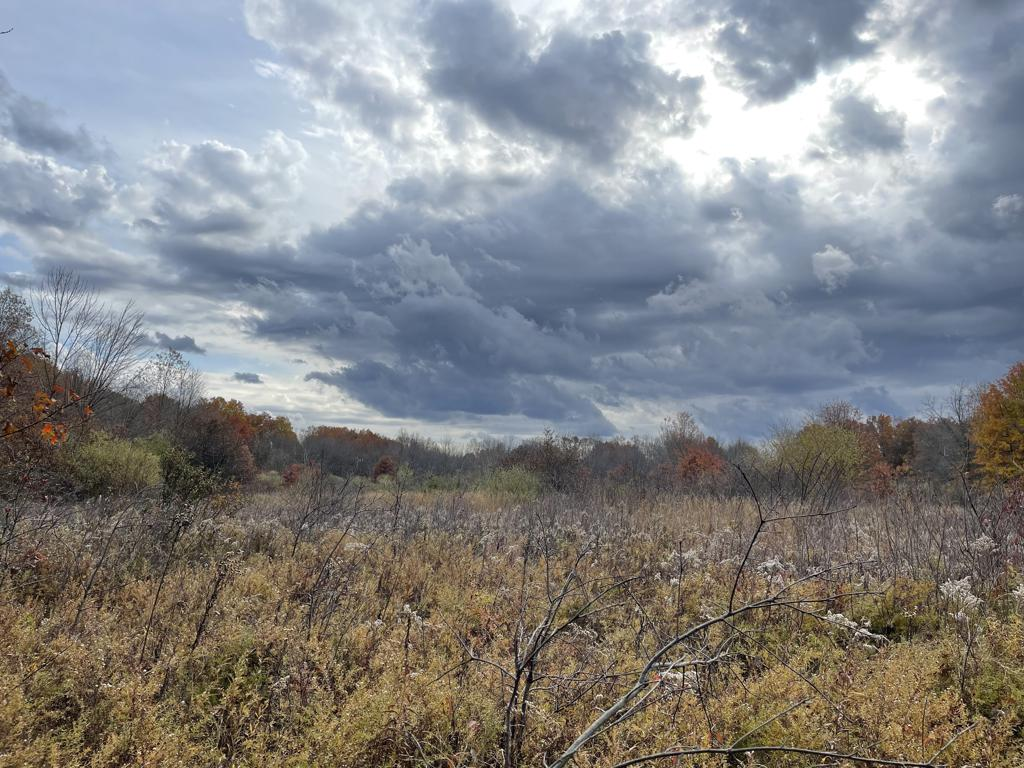2021-11-24

• Fat Cell

I found this photo of a fat cell that a food scientist had given me.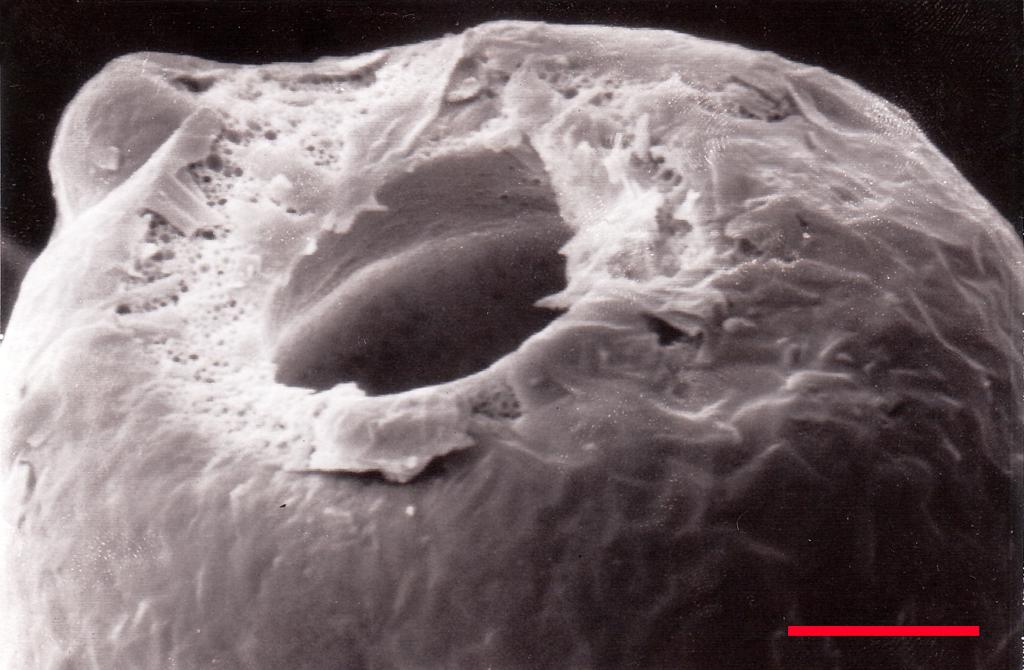I did some searching and quite frankly, I believe this to be one of the highest quality fat cell photos on the internet.

2021-05-04

• L-Systems

A weed had sprung up in a flower pot on a roof deck we once had. I watched it for a few weeks. It grew straight up and put out a few leaves. I wondered how high it would go and what would happen at the top. It required nothing from me. When it was tall enough to cast them wide, it grew a single flower and let the wind shake its seeds out. I liked that weed.

The image of weed-1 in Gary William Flake's The Computational Beauty of Nature reminded me of it.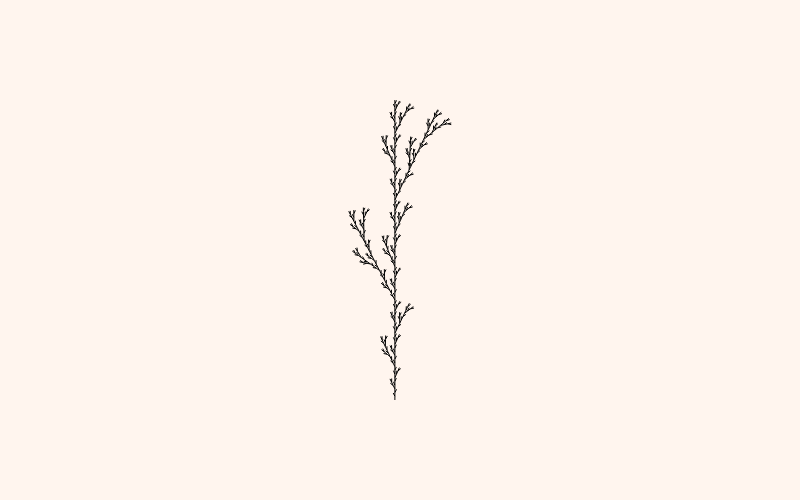I had been introduced to l-systems briefly by R. Luke Dubois in a computational music course and reading through Flake's description and list of rules, I thought: here is something fun I can make in less than an hour. I thought, the application of rules is straight forward (this was mostly true) and <Canvas/> element's 2D api is perfect for turtle graphics. I can use translate to move around, rotate to handle the turns and scale to make the image fit the canvas. I decided to use just the Flake text as a spec and got started. Of course, there were a few things that made it take a little longer.

L-Systems

L-Systems were invented by a botanist named Lindenmeyer to model plant growth. You start with an axiom and a set of rules and you apply the rules over a few generations. The resulting string is a set of drawing instructions. Weed-1 looks like this:

axiom: F

rule: F=F[-F]F[+F]F

This means start with F, everywhere you see an F, replace it with the string on the left hand side of the of equals mark. Apply that rule on the output a few times. These strings get big fast. Here's the resulting instructions for the weed show above (just three levels deep):

 1 2 3 4 5 6 7 8 9 10 11 12 13 14 15 16 17 18 19 20 21 22 23 24 25 26 27 F[-F]F[+F]F[-F[-F]F[+F]F]F[-F]F[+F]F[+F[-F]F[+F]F]F[-F]F[+F ]F[-F[-F]F[+F]F[-F[-F]F[+F]F]F[-F]F[+F]F[+F[-F]F[+F]F]F[-F] F[+F]F]F[-F]F[+F]F[-F[-F]F[+F]F]F[-F]F[+F]F[+F[-F]F[+F]F]F[ -F]F[+F]F[+F[-F]F[+F]F[-F[-F]F[+F]F]F[-F]F[+F]F[+F[-F]F[+F] F]F[-F]F[+F]F]F[-F]F[+F]F[-F[-F]F[+F]F]F[-F]F[+F]F[+F[-F]F[ +F]F]F[-F]F[+F]F[-F[-F]F[+F]F[-F[-F]F[+F]F]F[-F]F[+F]F[+F[- F]F[+F]F]F[-F]F[+F]F[-F[-F]F[+F]F[-F[-F]F[+F]F]F[-F]F[+F]F[ +F[-F]F[+F]F]F[-F]F[+F]F]F[-F]F[+F]F[-F[-F]F[+F]F]F[-F]F[+F] F[+F[-F]F[+F]F]F[-F]F[+F]F[+F[-F]F[+F]F[-F[-F]F[+F]F]F[-F]F[ +F]F[+F[-F]F[+F]F]F[-F]F[+F]F]F[-F]F[+F]F[-F[-F]F[+F]F]F[-F] F[+F]F[+F[-F]F[+F]F]F[-F]F[+F]F]F[-F]F[+F]F[-F[-F]F[+F]F]F[- F]F[+F]F[+F[-F]F[+F]F]F[-F]F[+F]F[-F[-F]F[+F]F[-F[-F]F[+F]F] F[-F]F[+F]F[+F[-F]F[+F]F]F[-F]F[+F]F]F[-F]F[+F]F[-F[-F]F[+F] F]F[-F]F[+F]F[+F[-F]F[+F]F]F[-F]F[+F]F[+F[-F]F[+F]F[-F[-F]F[ +F]F]F[-F]F[+F]F[+F[-F]F[+F]F]F[-F]F[+F]F]F[-F]F[+F]F[-F[-F] F[+F]F]F[-F]F[+F]F[+F[-F]F[+F]F]F[-F]F[+F]F[+F[-F]F[+F]F[-F[ -F]F[+F]F]F[-F]F[+F]F[+F[-F]F[+F]F]F[-F]F[+F]F[-F[-F]F[+F]F[ -F[-F]F[+F]F]F[-F]F[+F]F[+F[-F]F[+F]F]F[-F]F[+F]F]F[-F]F[+F] F[-F[-F]F[+F]F]F[-F]F[+F]F[+F[-F]F[+F]F]F[-F]F[+F]F[+F[-F]F[ +F]F[-F[-F]F[+F]F]F[-F]F[+F]F[+F[-F]F[+F]F]F[-F]F[+F]F]F[-F] F[+F]F[-F[-F]F[+F]F]F[-F]F[+F]F[+F[-F]F[+F]F]F[-F]F[+F]F]F[- F]F[+F]F[-F[-F]F[+F]F]F[-F]F[+F]F[+F[-F]F[+F]F]F[-F]F[+F]F[- F[-F]F[+F]F[-F[-F]F[+F]F]F[-F]F[+F]F[+F[-F]F[+F]F]F[-F]F[+F] F]F[-F]F[+F]F[-F[-F]F[+F]F]F[-F]F[+F]F[+F[-F]F[+F]F]F[-F]F[+ F]F[+F[-F]F[+F]F[-F[-F]F[+F]F]F[-F]F[+F]F[+F[-F]F[+F]F]F[-F] F[+F]F]F[-F]F[+F]F[-F[-F]F[+F]F]F[-F]F[+F]F[+F[-F]F[+F]F]F[- F]F[+F]F

The drawing instructions are interpreted as follows:

• F: Draw forward
• G: Move forward without drawing
• -: Turn left a specified angle
• +: Turn right a specified angle
• [: Push the current angle and position onto a FIFO stack
• ]: Pop the current angle and position from a FIFO stack
• |: Draw forward, but a shorter amount depending on your current depth

There are some other nuances (e.g. a number before a rotation means that you multiply that rotation by the number) but this is the general idea. There is plenty written about them online.

Application of rules

This part was mostly straight forward. Start with the axiom, loop over the rules, use a find an replace until you reach the desired depth.

 1 2 3 4 5 6 7 8 9 10 11 12 13 14 15 16 17 18 19 function run(axiom, rules, options) { let rule let transformation = axiom; let result = axiom; let depth = 0 let search, sub while (depth <= options.depth) { rules.forEach((rule) => { let [search, sub] = rule.split("=") axiom = axiom.replaceAll(search, sub) axiom = axiom.replaceAll(/(^|\D)\|/g, '\$1' + (depth) +'|') }) depth++ } return axiom }

As a bit of pre-rendering, I replace the | command with an integer at the current depth. This is the easiest way I could think to handle these.

Interpretation of rules

At first I thought I would just loop through the instructions:

• Move to some starting position near the center towards the bottom of canvas.
• Use the canvas path api to draw a line straight up a distance x.
• Move up x by translating.
• If I had to push or pop state, I could just use save() and restore() calls.
• When it came time to rotate, I would rotate().

It seemed the canvas's 2D drawing api had a method that matched each instruction, and that made sense. The canvas api and turtle graphics have a shared history.

But as I started I ran into a few shortcomings and suddenly this very simple solution fell apart.

Rotation

If you're performing the actions immediately as you interpret the commands you will run into problems with rotation. Assume you have three commands, move forward, rotate to the left and then move forward again.

• You encounter the F command so you draw a line up using lineTo
• You encounter the [ command so you rotate by calling rotate()
• You encounter another F command so you draw a line up using lineTo

You find that the lines won't connect, because rotate() rotates the entire coordinate system. In order to draw the line rotated you need to stop when you come to the rotate command, find the next line's center, translate to that point, then rotate and then translate back. Because the look ahead might not just be single step it could be tricky to figure out how far ahead to look.

The simple 1:1 application of commands to canvas api calls suddenly starts to show its rough edges.

Scaling

It also would have been nice to simple use the scale() call to shrink or expand my entire drawing to fill the space of the canvas. But doing this meant that my line width would scale as well. If I wanted to use scale() I would need to dynamically adjust this to compensate.

2D Vector Operations

My fall back was to process the commands into a series of 2D points, using simple 2D vector operations (rotate, add) to help rotate and navigate around the surface. I ended up liking this much better—the entire pipeline is basically a set of transformations on simple data structures (strings, lists of vectors, e.g.) and that gives me extensibility and flexibility.

The pipeline looks like this: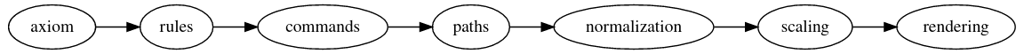The paths step is generated by starting at (0,0) and adding a vector (0,1) when we need to move forward. A rotation is maintained and the (0,1) vector is rotated before the addition happens. The result is an array of arrays. For instance the commands FF would result in:

 1 [[(0,0), (0,1), (0,2)]]

Once all the individual paths are generated, its easy to loop over them and divide them so they exist in a space between 0 and 1.

 1 2 3 4 5 6 7 8 9 10 11 12 13 14 15 16 17 18 19 20 21 22 23 function normalize(paths) { let [topLeft, bottomRight] = bounds(paths) let diff = (new Vector2d(0,0)).sub(topLeft); // make topLeft = 0,0 paths.forEach((path) => { path.forEach((point) => { point.add(diff) }) }) // set everything to range of 0 -> 1 let [tl, br] = bounds(paths) let divisor = Math.max(tl.x, br.y); paths.forEach((path) => { path.forEach((point) => { point.div(divisor) }) }) return paths; }

So the above would become:

 1 [[(0,0), (0,.5), (0,1)]]

From there, it's easy to scale these paths to any dimensions by looping through the vectors and calling a scale() method on them. All the hard work is down by the vector datatype (the type of point below).

 1 2 3 4 5 6 7 8 function scale(paths, scale) { paths.forEach((path) => { path.forEach((point) => { point.scale(scale) }) }) return paths }

Rendering

With the data structure being a simple list of points, rendering is easy. To render in SVG, just loop through and create a long path string:

 1 2 3 4 5 let svgpath = paths.reduce((carry, path) => { let first = path.shift() let move = " M " + first.x + " " + first.y + " " + path.reduce((carry, pos) => carry + " L " + pos.x + " " + pos.y , "") return carry + move; }, "");

Resulting in something like the following for weed-1:

 1 M 42.43528234040036 300 L 40.96059540786641 292.898838485544 M 42.43528234040036 296.2962962962963 L 44.01807463861047 289.2441318020867

To render in canvas just use the moveTo and lineTo calls:

 1 2 3 4 5 6 7 8 9 ctx.beginPath() paths.forEach((path) => { let start = path.shift() ctx.moveTo(start.x, start.y) path.forEach((point) => { ctx.lineTo(point.x, point.y) }) }) ctx.stroke()

2021-04-05

2021-03-25

• There Are Bugs in the Gonis

A former colleague of mine once shared a picture of some sentences that one of his wife's student's had written. The student was a very young kid with very young kid handwriting and the sentences were funny and we all had some laughs at how cute they were.

One of the sentences was partly incomprehensible. As far as we could tell it looked like it said "There are bugs in the gonis." For some reason this was hilarious to us and because we all wrote software for a job we began to use the word "gonis" to refer to any complex, old or difficult-to-work-with piece of code: it's where bugs come from.

I don't work there anymore. Neither does that colleague. And his wife no longer teaches those hilarious kids. But not a week goes by that I don't find myself at my new job wishing I could call some file or system a gonis.

So I figured I would write this little story. That way I can just use the word freely and hope that people drop it into a search engine and find this.

2021-03-09

see all